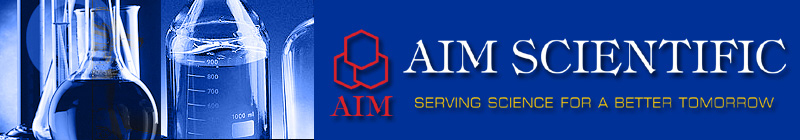• Teaching Learning Material
• Teacher Resource Kit
• Number Balance
• Hook N Look Numerical Balance
• Number Planet Kit
• Maths Learning Kit
• Vernier Callipers
• Dummy Clock
• Model of Screw Gauge
• Model of Vernier Calliper
• Hollow Cylinder
• Ring of Theorem
• Combination of Cube & Sphere
• Magnetic Base Ten Blocks
• Tangram
• Abacus
• Abacuses
• Clock Wooden Model
• Count 0-9
• Count & Match
• Flash Cards
• Geoboard Round
• Geoboard Square
• Mental Math Puzzle
• Puzzle Our Body
• Spike Abacus
• Volume Set
• Geometrical Shapes
• Kitchen Scale
• Theodolite Model
• Wooden Scale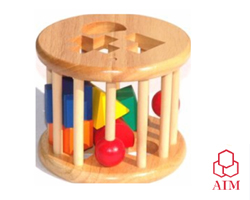Teaching Learning Material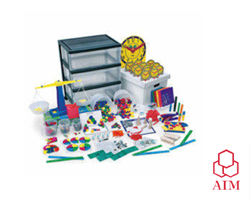Teacher Resource Kit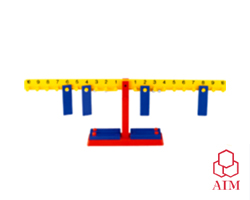Number Balance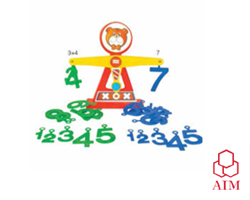Hook N Look Numerical Balance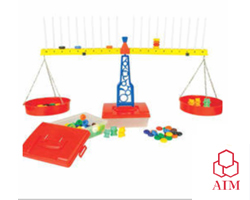Number Planet Kit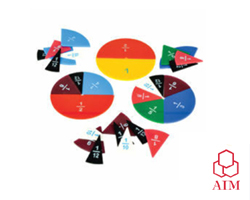Maths Learning Kit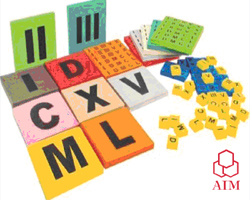Roman Number Kit For Mathematics Kit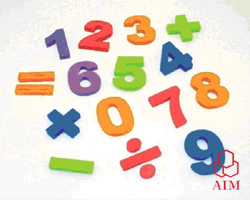Number with Plate For Mathematics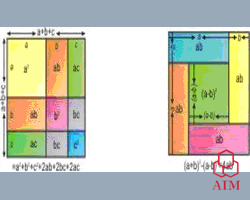Algebra Identity For Mathematics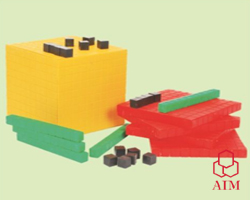Base and Place Value Kit For Mathematics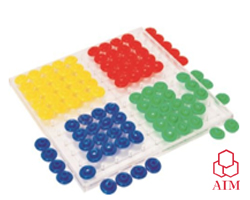Power of Two Math Lab Item For MathematicsAngle Property of Cyclic Quadriliteral For Math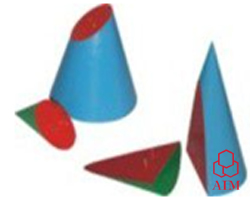Conic section For Mathematics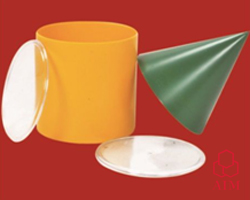Volume Relation between Cone and Cylinder For MathTriangle Kit For Mathematics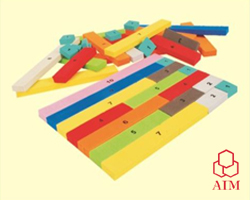Cusinaire Strips For MathematicsPythagorus Theorem For Mathematics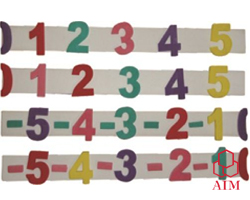Integer number line Bar For Mathematics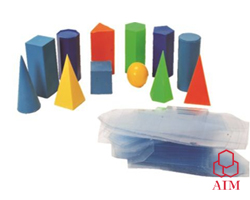Polyhedron And Their Net For Mathematics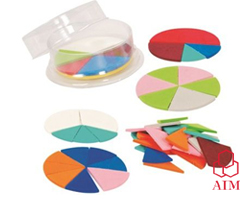Fraction Wheel For Mathematics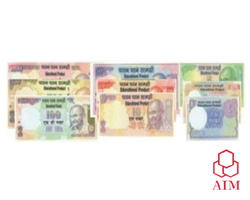Dummy Currency Notes For Mathematics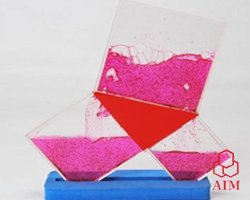Working Model of Pythagorus theorem Mathematics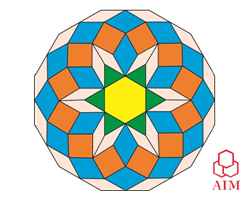Educational Pattern Blocks For Mathematics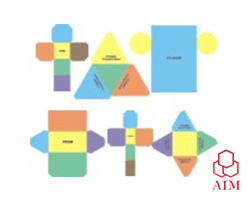Paper nets of Solid Shapes For Mathematics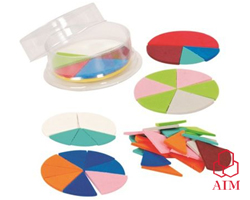Fraction Wheel EVA Foam For Mathematics1 CM Interlocking Cubes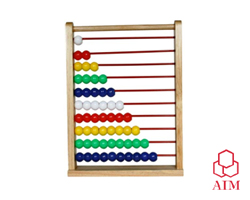Wooden AbacusStencils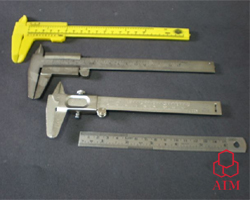Vernier Callipers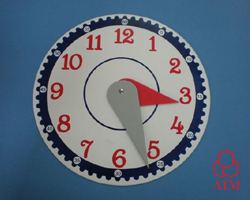Dummy Clock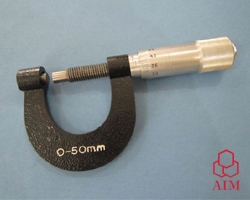Model of Screw Gauge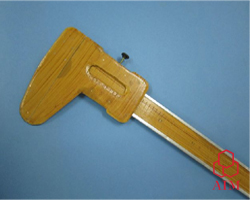Model of Vernier Calliper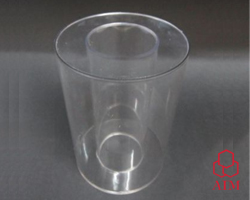Hollow Cylinder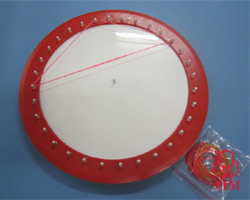Ring of Theorem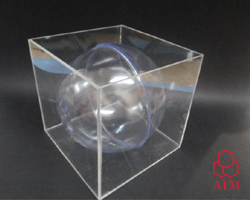Combination of Cube & Sphere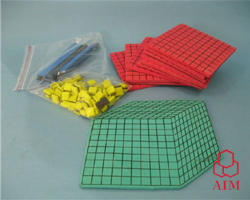Magnetic Base Ten Blocks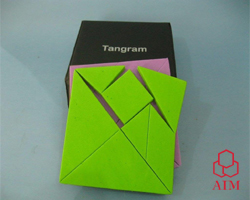Tangram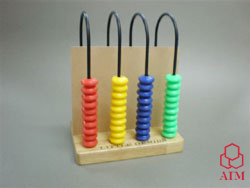Abacus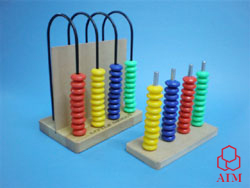Abacuses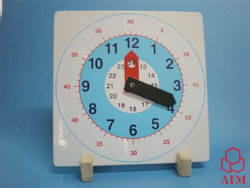Clock Wooden Model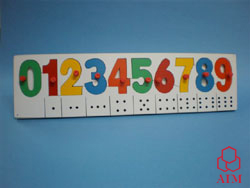Count 0-9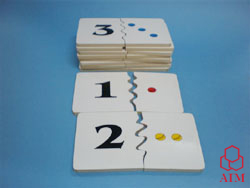Count & Match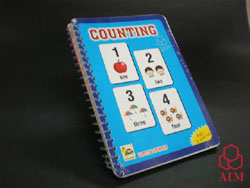Flash Cards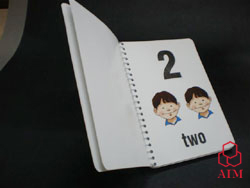Flash Cards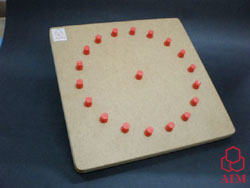Geoboard Round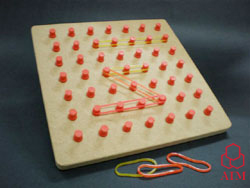Geoboard SquareMental Math Puzzle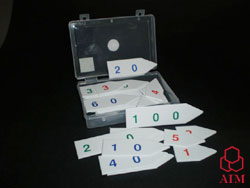Puzzle Our Body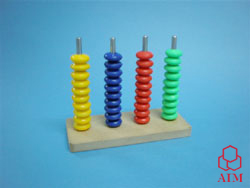Spike Abacus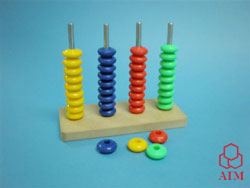Spike Abacus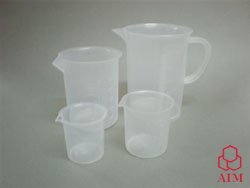Volume SetGeometrical Shapes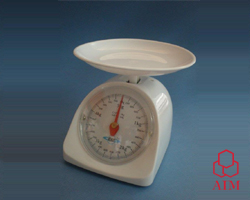Kitchen Scale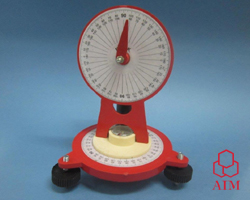Theodolite Model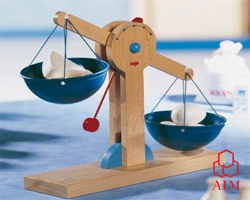Wooden Scale

 NOTE:- The information and specification provided are subject to change without notice. © Copyright : AIM SCIENTIFIC : 457, F.F., F.I.E., PATPARGANJ INDUSTRIAL AREA, NEW DELHI - 110092 (INDIA) Tel :+91-11-43054884, 7042444061, 41354884 E-mail : info@aimscientific.com